# Procedure: Fire a practice shot. Locate the landing point on the floor then secure a piece...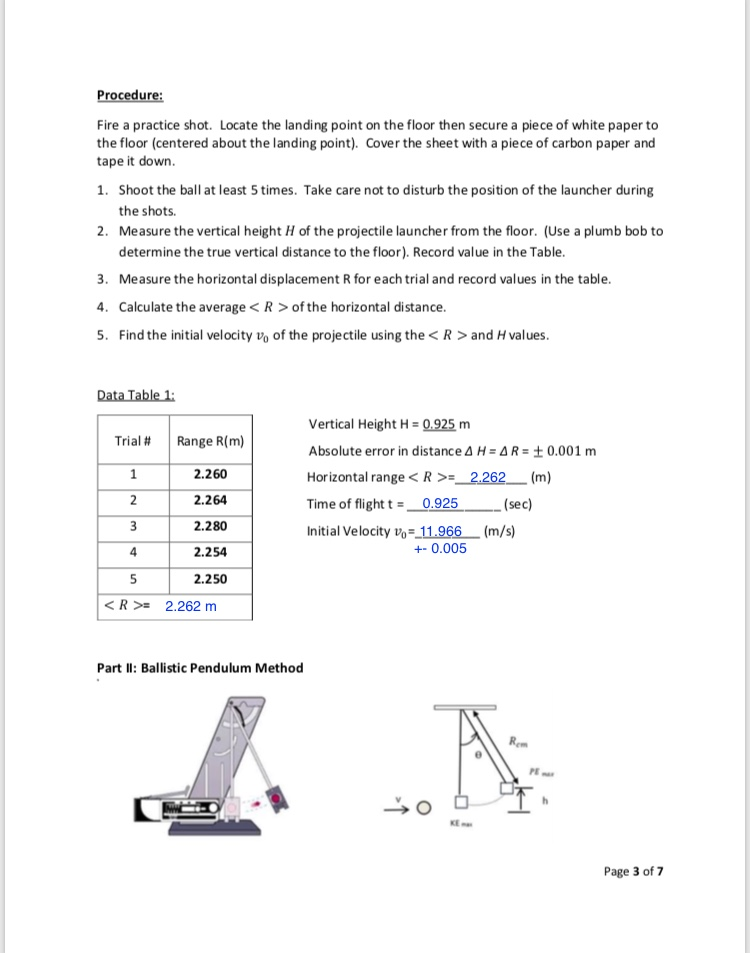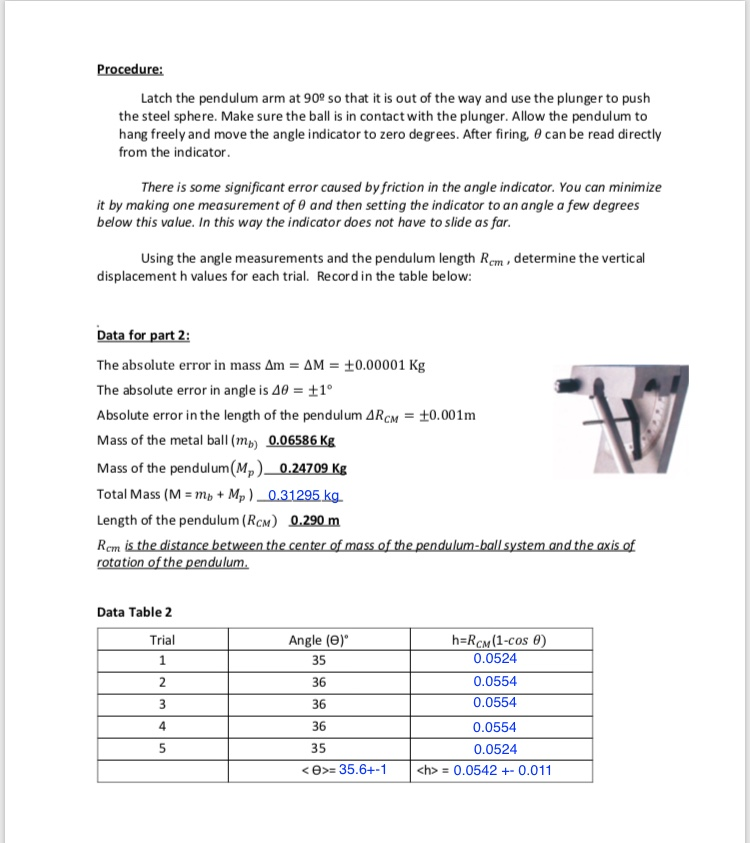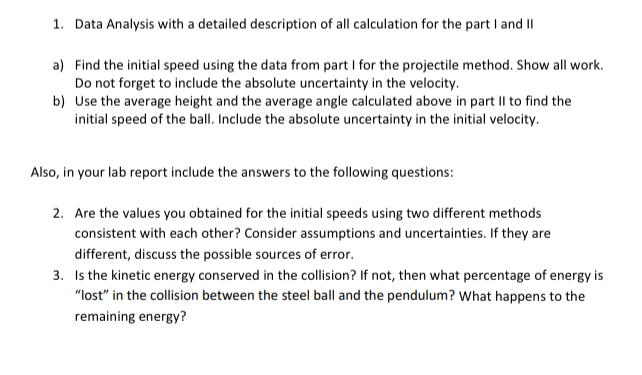Procedure: Fire a practice shot. Locate the landing point on the floor then secure a piece of white paper to the floor (centered about the landing point). Cover the sheet with a piece of carbon paper and tape it down. 1. Shoot the ball at least 5 times. Take care not to disturb the position of the launcher during the shots. 2. Measure the vertical height H of the projectile launcher from the floor. (Use a plumb bob determine the true vertical distance to the floor). Record value in the Table. 3. Measure the horizontal displacement R for each trial and record values in the table. 4. Calculate the average <R> of the horizontal distance. 5. Find the initial velocity vo of the projectile using the < R > and H values. Data Table 1: Trial # Range R(m) 1 2.260 2.264 Vertical Height H = 0.925 m Absolute error in distance 4 H = AR = 0.001 m Horizontal range <R>=__2.262_(m) Time of flight t = _0.925 (sec) Initial Velocity v=-11.966 (m/s) +-0.005 2 3 2.280 4 2.254 5 2.250 <R>= 2.262 m Part II: Ballistic Pendulum Method yo Page 3 of 7
Procedure: Latch the pendulum arm at 909 so that it is out of the way and use the plunger to push the steel sphere. Make sure the ball is in contact with the plunger. Allow the pendulum to hang freely and move the angle indicator to zero degrees. After firing, can be read directly from the indicator There is some significant error caused by friction in the angle indicator. You can minimize it by making one measurement of and then setting the indicator to an angle a few degrees below this value. In this way the indicator does not have to slide as far. Using the angle measurements and the pendulum length Rem, determine the vertical displacement h values for each trial. Record in the table below: Data for part 2: The absolute error in mass Am = AM = +0.00001 Kg The absolute error in angle is 40 = +1° Absolute error in the length of the pendulum ARCM = +0.001m Mass of the metal ball (mp) 0.06586 Kg Mass of the pendulum(M) 0.24709 Kg Total Mass (M = mp+Mp)_ 0.31295 kg Length of the pendulum (Rcm) 0.290 m Rem is the distance between the center of mass of the pendulum-ball system and the axis of rotation of the pendulum. Data Table 2 Trial Angle (0) 35 1 2 3 h=Rcm (1-сos ) 0.0524 0.0554 0.0554 0.0554 0.0524 <h> = 0.0542 +-0.011 36 36 36 35 <>= 35.6+-1 4 5
1. Data Analysis with a detailed description of all calculation for the part I and II a) Find the initial speed using the data from part I for the projectile method. Show all work. Do not forget to include the absolute uncertainty in the velocity. b) Use the average height and the average angle calculated above in part II to find the initial speed of the ball. Include the absolute uncertainty in the initial velocity. Also, in your lab report include the answers to the following questions: 2. Are the values you obtained for the initial speeds using two different methods consistent with each other? Consider assumptions and uncertainties. If they are different, discuss the possible sources of error. 3. Is the kinetic energy conserved in the collision? If not, then what percentage of energy is "lost" in the collision between the steel ball and the pendulum? What happens to the remaining energy?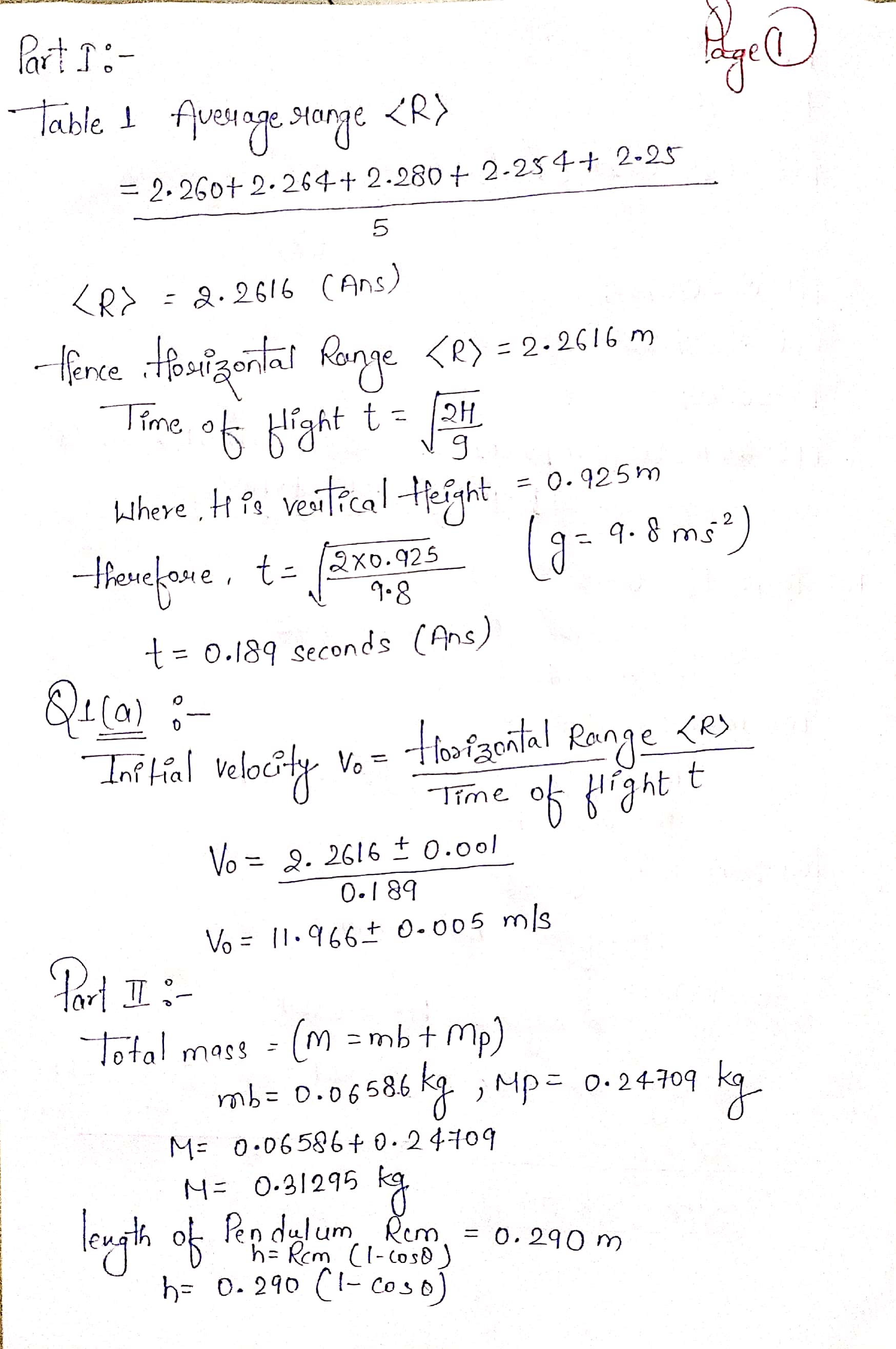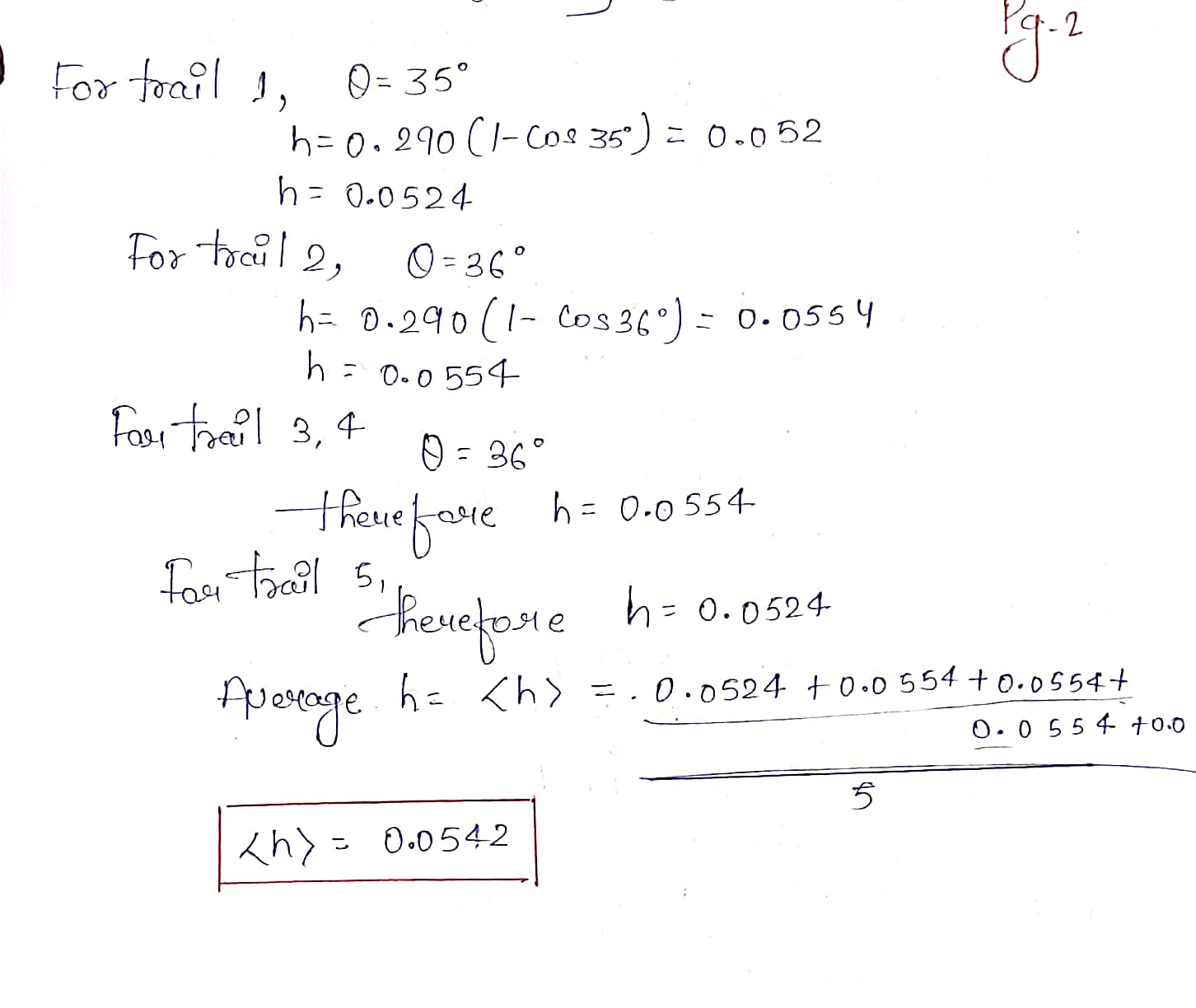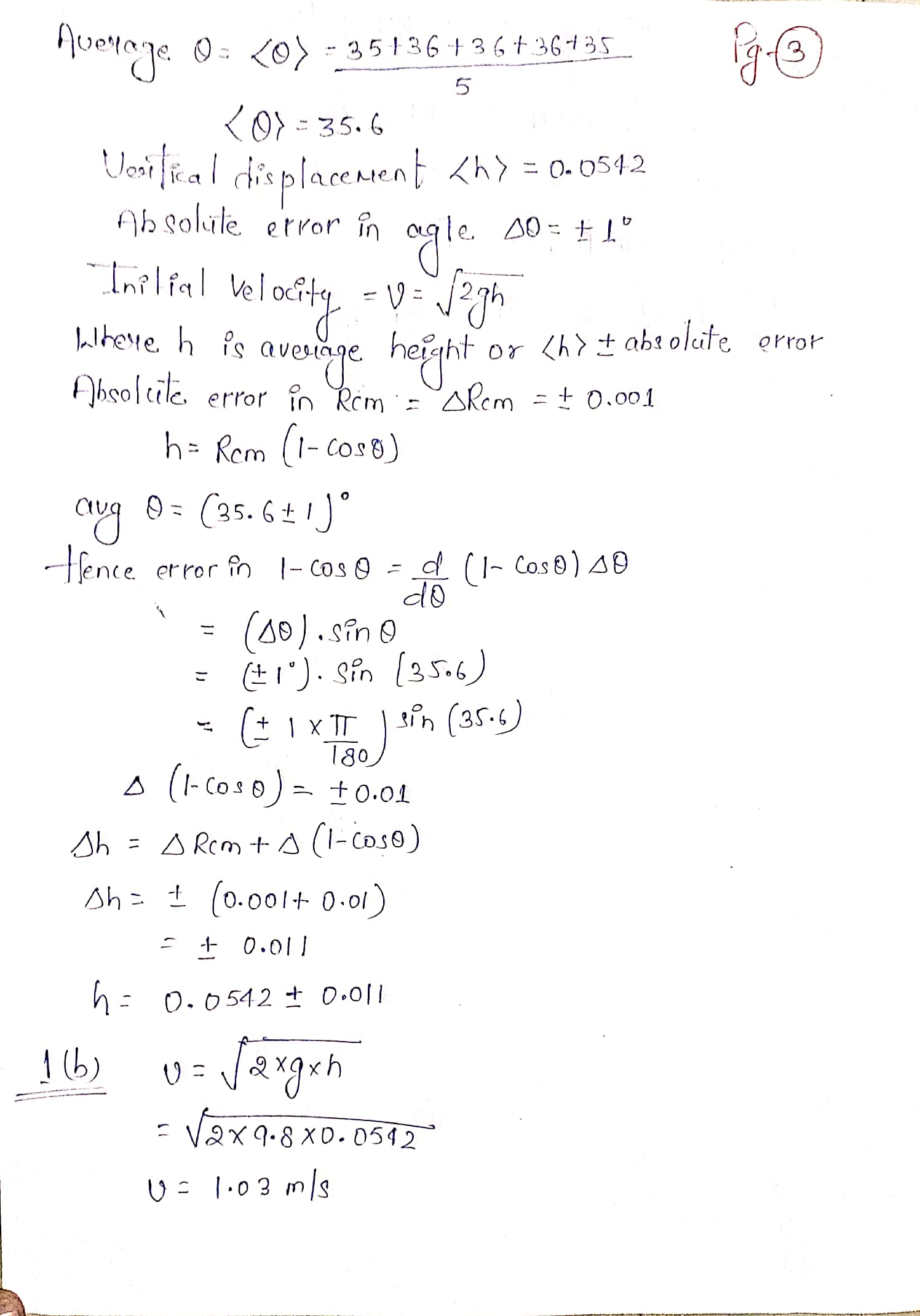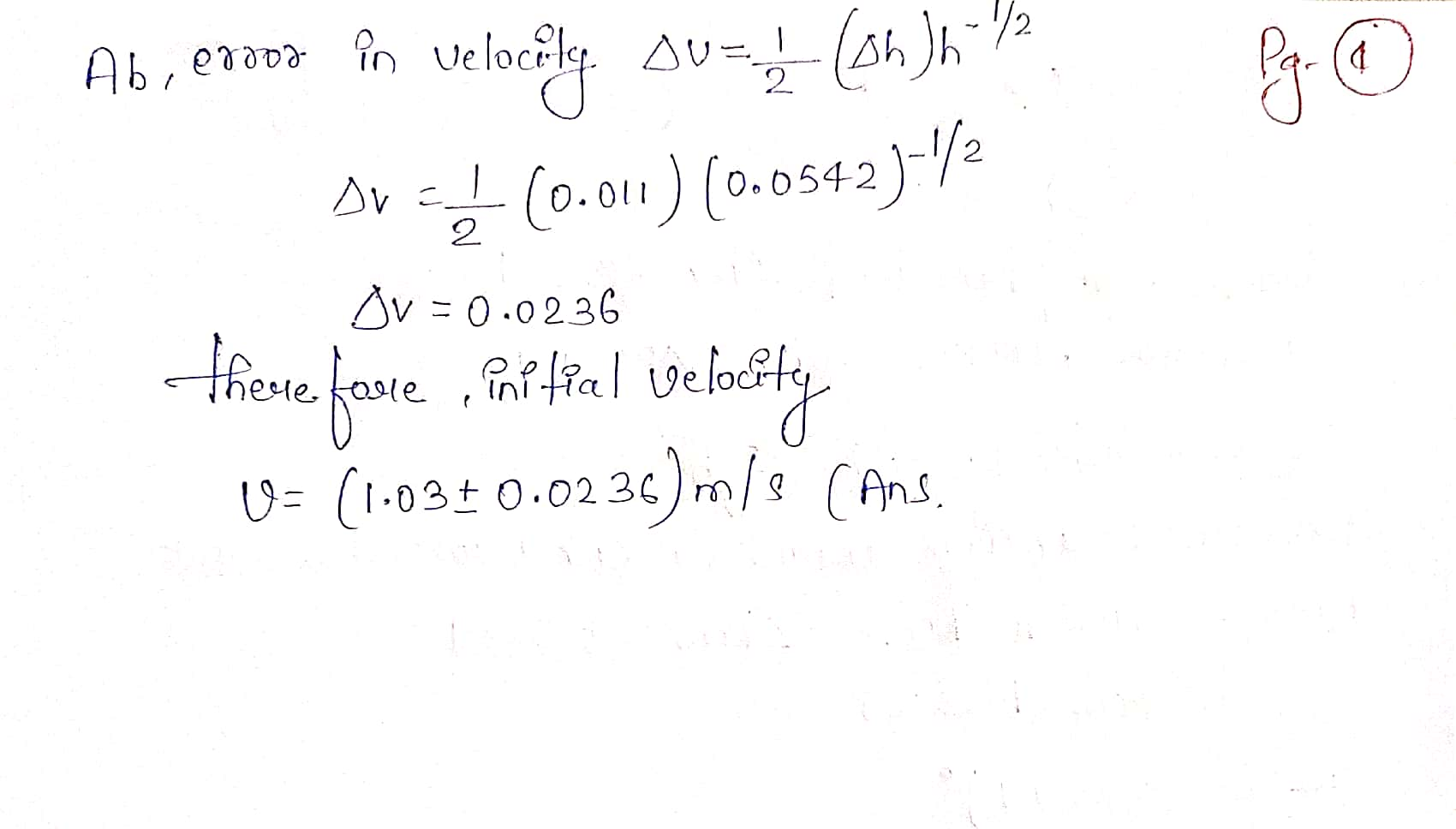Note:please don't give dislike .......please comment if you have any problem i will try to solve your problem...... please give thumbs up i am in need....

#### Earn Coin

Coins can be redeemed for fabulous gifts.

Similar Homework Help Questions
• ### Ballistic Pendulum Version Procedure: Fire a practice shot. Locate the landing point on the floor then...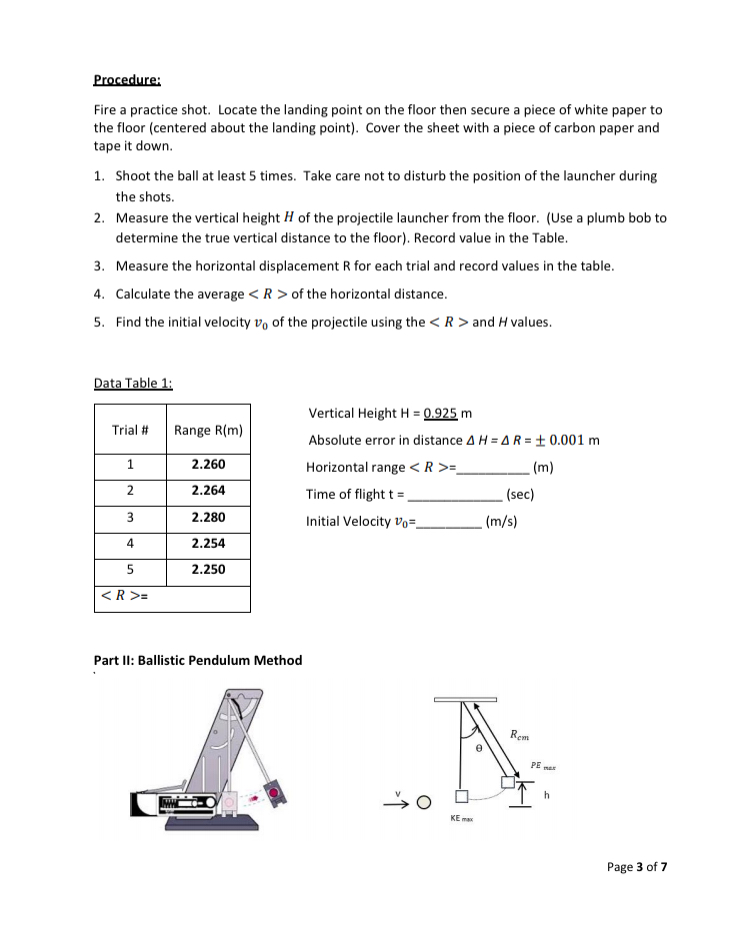Ballistic Pendulum Version Procedure: Fire a practice shot. Locate the landing point on the floor then secure a piece of white paper to the floor (centered about the landing point). Cover the sheet with a piece of carbon paper and tape it down. 1. Shoot the ball at least 5 times. Take care not to disturb the position of the launcher during the shots. 2. Measure the vertical height H of the projectile launcher from the floor. (Use a plumb...

• ### Part b & c Name Date Data Sheet Experiment 21: Ballistic Pendulum A. The Ballistic Pendulum...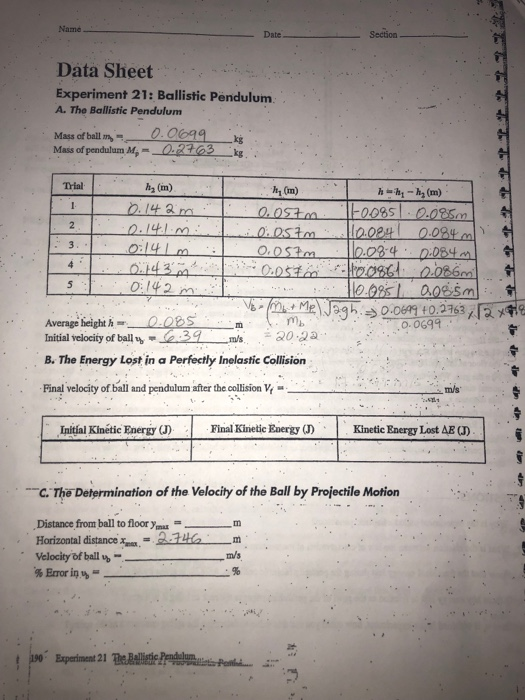Part b & c Name Date Data Sheet Experiment 21: Ballistic Pendulum A. The Ballistic Pendulum Mass of ball m- Mass of pendulum M,-0.23 k Trial h2 (m) h1 (m) 0 0:142 Average heighthORSm Initial velocity of ball2ms 30 3 B. The Energy Lost in a Perfectly Inelastic Collision mb 0.0699 Final velocity of ball and pendalam aer the collision m/s Initial Kinétic Energy (J) ie Eaergy ) Kinetic Energy Lost AB a) C.The Determination of the Velocity of the...

• ### TRIAL 1 600 2.610 9.91 o.2 0.39 2.62 43 Average Result: The average speed of the given ball is ve...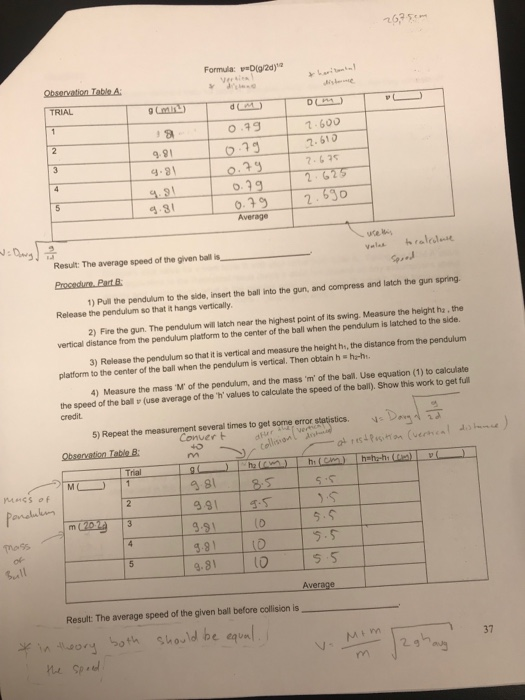TRIAL 1 600 2.610 9.91 o.2 0.39 2.62 43 Average Result: The average speed of the given ball is velas hralels 1) Pull the penduum to the side, insert the ball into the gun, and compress and latch the gun spring Release the pendulum so that it hangs vertically 2) Fire the gun. The pendulum will latch near the highest point of its swing. Measure ne height ha·the vertical distance from the pendulum platform to the center of the ball...

• ### Determining the Initial Velocity ) Vertical Distance O15 Horizontal Distance to paper edge 2 05 m...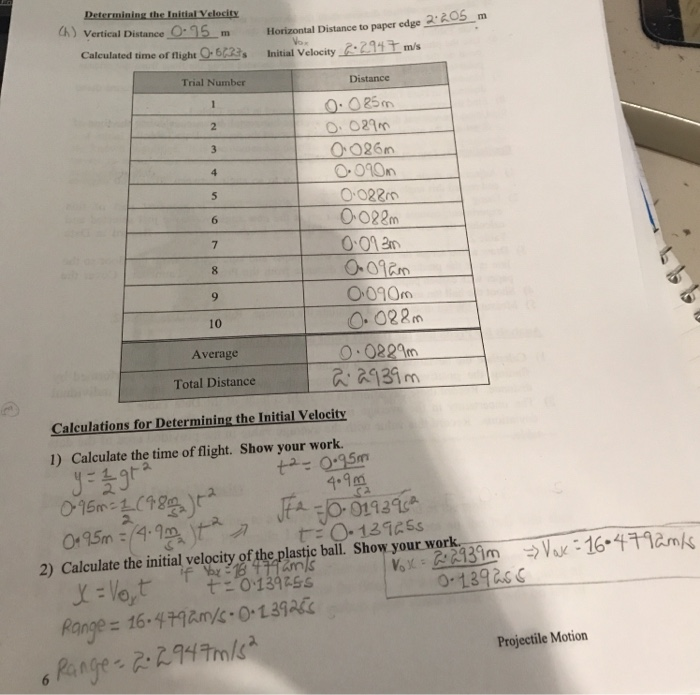Determining the Initial Velocity ) Vertical Distance O15 Horizontal Distance to paper edge 2 05 m V Calculated time of flight O623s Initial Velocity-2947 m/s Trial Number Distance 1 O029m 3 O0260 4 5 O022m O022m 6 7 O090m O. 022m O.0229m 939m 9 10 Average Total Distance Calculations for Determining the Initial Velocity 1) Calculate the time of flight. Show your work taz0.95m 4.9m sa 2 O4 95m-4 -1 jta t0-12925s 2) Calculate the initial velocity of the plastic...

• ### Need help With Analysis questions. Ballistic Pendulum Lab In this lab, you will explore conservat...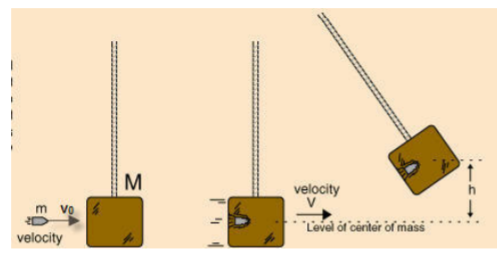Need help With Analysis questions. Ballistic Pendulum Lab In this lab, you will explore conservation of Energy and Momentum in an inelastic collision between a projectile and a cardboard box suspended from a string. In particular, you will see that the transfer of linear momentum is easily accounted for in an inelastic collision, but the transfer of kinetic energy is not easy to measure directly. This is because Kinetic Energy in collisions transfers to vibrational, acoustic, and potential energy. Once...

• ### A ball is projected horizontally from the edge of a table that is 4.0 meters high, and strikes the floor at a point 3.0 meters from a point on the floor directly below the edge of the table

1. A ball is projected horizontally from the edge of a table that is 4.0 meters high, and strikes the floor at a point 3.0 meters from a point on the floor directly below the edge of the table. The initial velocity of the ball as it is projected from the table is, in m/s ( g=9.8 m/s2) 2. At an airport, luggage is loaded into two cars of a luggage carrier being pulled by a luggage motocart whose mass...

• ### Name Date Section Data Sheet Experiment 21: Ballistic Pendulum. A. The Ballistic Pendulum Mass ofball m-9.890....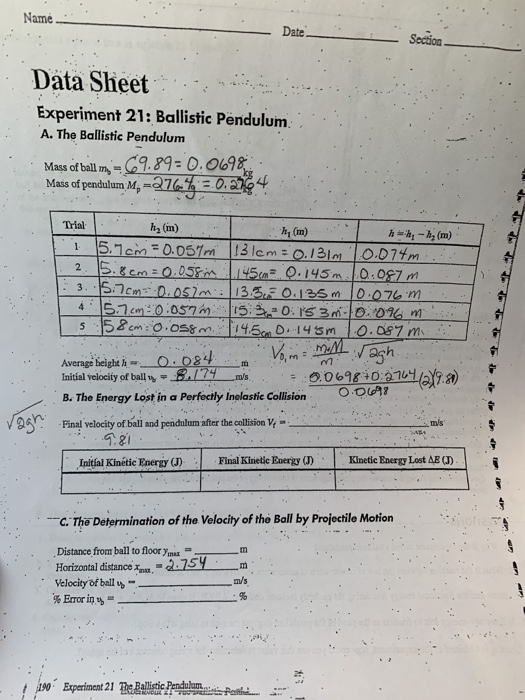Name Date Section Data Sheet Experiment 21: Ballistic Pendulum. A. The Ballistic Pendulum Mass ofball m-9.890. 0698 Mass of pendulum Mp- Trial h2 (m) hy (m) 4.1らコcm : 0.057 in 115.220. IS 3m-10.01am Average beight h Initial velocity of ballm's B. The Energy Lost in a Perfocily Inolastic Collsion O 00 698 01a nal velodic mis Initíal Kinétic Energy (J) Final Kinetic EnergyKinetic Energy Lost AB C. The Determination of the Velocity of the Ball by Projectile Motion Distance from...

• ### Derive the expression for the initial velocity v of a ballistic pendulum in terms of the...

Derive the expression for the initial velocity v of a ballistic pendulum in terms of the swept-out angle theta. Use the following equations of momentum and energy conservation... When the projectile and the pendulum stick together, they have total mass m + M and we define their combined velocity to be V⃗ . The relationship from conservation of momentum is: mv = (m + M)V⃗ Conservation of energy requires the initial kinetic energy of the pendulum system to equal the...

• ### THE EXPERIMENT The speed "." of a ball as it leaves the spring gun will be...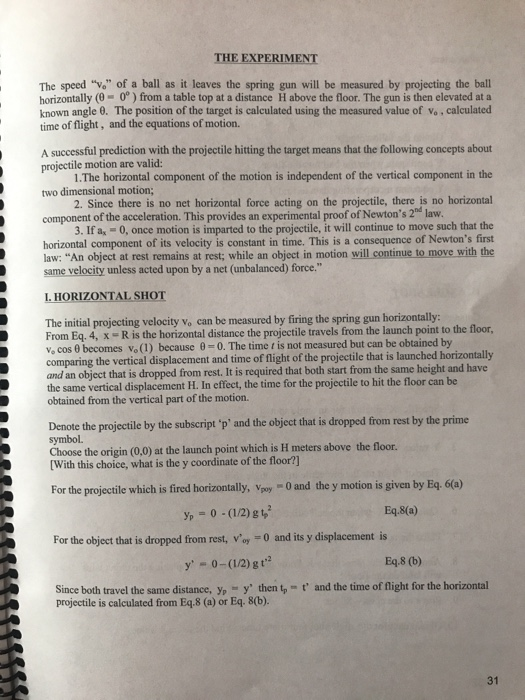THE EXPERIMENT The speed "." of a ball as it leaves the spring gun will be measured by projecting the ball horizontally (0-0) from a table top at a distance H above the floor. The gun is then elevated at a known angle 8. The position of the target is calculated using the measured value of v. calculated time of flight, and the equations of motion. A successful prediction with the projectile hitting the target means that the following concepts...

• ### Tx= vo2/g Sin20 (0 with line through the middle) where Tx= horizontal range, vo= initial velocity,...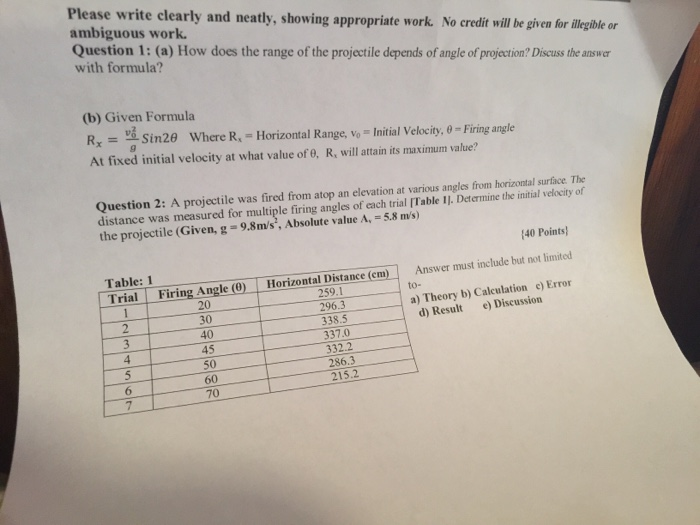Tx= vo2/g Sin20 (0 with line through the middle) where Tx= horizontal range, vo= initial velocity, o firing angle at fixed velocity. At which value of 0, r, will attain its maximum value? Please write clearly and neatly, showing appropriate work. No credit will be given for ambiguous work Question 1: (a) How does the range of the projectile depends of angle of projection? Discuss the answer with formula? d neatly, showing appropriate work. No credit will be given for...